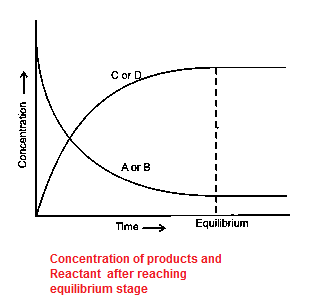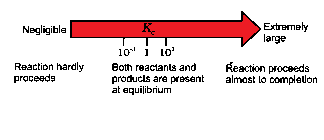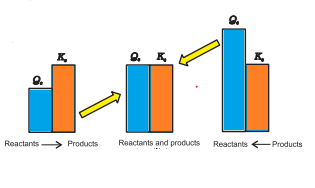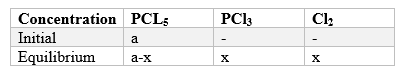# Chemical Equilibrium

## Chemical Equilibrium:-

• Chemical Equilibrium is defined as the equilibrium attained between reactants and products in the chemical reaction.
• These reactions occur both in forward and backward directions. When the rates of the forward and reverse reactions become equal, the concentrations of the reactants and the products remain constant. This is the stage of chemical equilibrium
• The equilibrium is dynamic in nature as the reaction is still happening in both forward and reverse direction

## Equilibrium mixture

The mixture of reactants are products is Equilibrium state is called Equilibrium mixture
Example
$N_2 + 2H_2 \rightleftharpoons 3NH_3$
At Equilibrium rate of forward reaction = Rate of background reaction
$r_f = r_b$
This can be generalized as
$A + B \rightleftharpoons C + D$
This can be visualized in the below graph also## Law of Mass Action or equibrium equation

$R \rightleftharpoons P$
Rate of forward reaction
$r_ f \propto [R]$
Rate of backward reaction
$r_b \propto [P]$
Equilibrium constant is defined as
$K_c = \frac {r_f}{r_b} = \frac {[P]}{[R]}$
In General form
$A + B \rightleftharpoons C + D$
$Reactants \rightleftharpoons Product$
$K_c =\frac {[C][D]}{[A][B]}$
KC = equilibrium constant
For
$aA + bB \rightleftharpoons cC + dD$
$K_c =\frac {[C]^c[D]^d}{[A]^a[B]^b}$
Definition of KC
It is defined as the ratio of product of products to the product of reactants raised to the power of stoichiometric constant
Examples
$N_2 (g) + 3H_2 (g) \rightleftharpoons 2NH_3 (g)$.
$K_c =\frac {[NH_3]^2}{[N_2][H_2]^3}$
$H_2 + I_2 \rightleftharpoons 2HI$
$K_c =\frac {[HI]^2}{[H_2][I_2]}$

## Important points for Equilibrium constants

1. When equilibrium is reversed the value of KC will get reciprocated.
Example
$H_2 + I_2 \rightleftharpoons 2HI$
$K_c =\frac {[HI]^2}{[H_2][I_2]}$
$2HI \rightleftharpoons H_2 + I_2$
${K_c}^1 =\frac {[H_2][I_2]}{[HI]^2}$
$K_c = \frac {1}{{K_c}^1}$
2. Where equation are added the value of KC will be multiplied.
Example
$M + L \rightleftharpoons ML$
$K_1 = \frac {[ML]}{[M][L]}$
$ML + L \rightleftharpoons ML_2$
$K_2 = \frac {[ML_2]}{[ML][L]}$
$ML_2 + L \rightleftharpoons ML_3$
$K_3 = \frac {[ML_3]}{[ML_2][L]}$
$M + 3L \rightleftharpoons ML_3$
$K_c=\frac {[ML_3]}{[M][L]^3}$
$K_c = K_1 \times K_2 \times K_3$
In general Form
$A \rightleftharpoons B$
$C \rightleftharpoons D$
$A + C \rightleftharpoons B + D$
So,
$K_3= K_1 \times K_2$
3. When equation is multiplied by n; then the value of KC is raised to the power n.
$A + B \rightleftharpoons C + D$
$K_c =\frac {[C][D]}{[A][B]}$
$nA + nB \rightleftharpoons nC + nD$
${K_c}^1 =\frac {[C]^n[D]^n}{[A]^n[B]^n}$
So,
${K_c}^1 = (K_c)^n$
4. Expression for equilibrium constant is applicable only when concentrations of the reactants and products have attained constant value at equilibrium state
5. The value of equilibrium constant is independent of initial concentrations of the reactants and products.
6. Equilibrium constant is temperature dependent having one unique value for particular reaction represented by a balanced equation at a given temperature.
Examples
1. $2SO_2 (g) + O_2 (g) \rightleftharpoons 2SO_3$
Calculate $K_c$ if [SO2] = 0.6 M; [O2] = 0.82 M [SO3] = 1.9 M
Solution
$K_c = \frac {[SO_3]^2}{[SO_2]^2[O_2]}$
=12.5
2. $N_2 + 3H_2 \rightleftharpoons 2NH_3$
[N2]= 0.1 M; [H2] = 0.1 M, [NH3 ]=.2 M
Solution
$K_c = \frac {[NH_3]^2}{[N_2][H_2]^3}$
=400

## Homogeneous Equilibrium

In a homogeneous system, all the reactants and products are in the same phase. For example,
$N_2 + 3H_2 \rightleftharpoons 2NH_3$
For gaseous state, we usually specify equilibrium constant in terms of partial pressure and it is denoted by Kp
for $H_2 + I_2 \rightleftharpoons 2HI$
$K_p = \frac {[p_HI]^2}{[p_I2][p_H2]}$
Relation between $K_p$ and $K_c$
$K_p=K_c$
for $N_2 + 3H_2 \rightleftharpoons 2NH_3$
$K_p = \frac {[p_NH3]^2}{[p_N2][p_H2]^3}$
Relation between $K_p$ and $K_c$
$K_p=K_c (RT)^-2$
In general
$aA + bB \rightleftharpoons cC + dD$
$K_p = \frac {[p_C]^c[p_D]^d}{[p_A]^a[p_B]^b}$
Relation between $K_p$ and $K_c$
$K_p=K_c (RT)^{\Delta n}$
where $\Delta n = c+d -(a+b)$

## heterogeneous Equilibrium

Equilibrium in a system having more than one phase is called heterogeneous equilibrium
For example
In closed vessel
$Ca CO_3 (s) \rightleftharpoons Ca O (s) + CO_2 (g)$
Heterogeneous equilibrium often involve pure solids or liquids. We can simplify equilibrium expressions for the heterogeneous equilibria involving a pure liquid or a pure solid, as the molar concentration of a pure solid or liquid is constant i.e
$[Ca CO_3]=1 ,[CaO]=1$
$K_c=[CO_2]$

## How to Predict the extent of the Reaction

KC is used to predict the magnitude of the reaction.if $K_c$ is very large, the reaction proceeds nearly to completion
if $K_c$ is very small, the reaction proceeds rarely
If $K_c$ is in the range of $10_{-3}$ and to $10_3$,appreciable concentrations of both reactants and products are present
Reaction Quotient:-

It is the ratio of molar concentration of products to the molar concentration of reactants at any time of the reaction.
QC is used when molar concentration is used
QP is used when partial pressure is used
If Q = KC i. e. reaction is at Equilibrium.
If Q > KC [Left side] i. e. reaction did not complete. The reaction will proceed in the direction of reactants (reverse reaction)
If Q < KC [Right side] i.e. reaction is almost complete.the reaction will proceed in the direction of the products (forward reaction).## How to calculate Equilibrium concentration

Step 1. First Write the balanced equation for the chemical reaction.
$PCl_5 \rightleftharpoons PCl_3 + Cl_2$
Step 2. For the balanced equation, make a table that lists for each substance involved in the reaction:
(a) the initial concentration, ( a)
(b) the change in concentration on going to equilibrium(x)
(c) the equilibrium concentration.
We can define x as the concentration (mol/L) of one of the substances that reacts on going to equilibrium, then use the stoichiometry coefficient of the reaction to determine the concentrations of the other substances in terms of x.Step 3. Substitute the equilibrium concentrations into the equilibrium equation for the reaction and solve for x. For quadratic equation choose the mathematical solution that makes chemical sense.
$K_c=\frac {[PCL_3][CL_2]}{]PCL5]}$
$K_c= \frac {x^2}{a-x}$
$x^2 +xK_c-aK_c=0$
Step 4. Now we can easily derive the value of the products and reactant at the equilibrium stage by putting the values of x
Example
if a = 3m/L and $K_c=1.8$
then
$x^2 +1.8x-5.4=0$

Solving the Quadratic Equation and ignoring negative value
x= 1.59
Then $[PCL_5]=3 -1.59 = 1.41$
and $[PCL_3]=[CL_2] = 1.41$

## Relation between Gibbs Energy (G) and KC

G ---- Gibb’s free energy
$\Delta G^{\ominus} = -RT ln K_c$
$\Delta G^{\ominus} = -2.303 RT log K_c$
Now
$K=e^{\frac {-\Delta G^{\ominus}}{RT}}$
If $\Delta G^{\ominus} < 0$, then K >1, which implies a spontaneous reaction or the reaction which proceeds in the forward direction to such an extent that the products are present predominantly. •
If $\Delta G^{\ominus} > 0$,, K < 1, which implies a non-spontaneous reaction or a reaction which proceeds in the forward direction to such a small degree that only a very minute quantity of product is formed.